# Linear Equations: Precalculus Lesson Plans Chapter Exam

Exam Instructions:

Choose your answers to the questions and click 'Next' to see the next set of questions. You can skip questions if you would like and come back to them later with the yellow "Go To First Skipped Question" button. When you have completed the practice exam, a green submit button will appear. Click it to see your results. Good luck!

### Page 1

#### Question 1 1. If the graph below has the equation Ax+By=C, which statement MUST be true?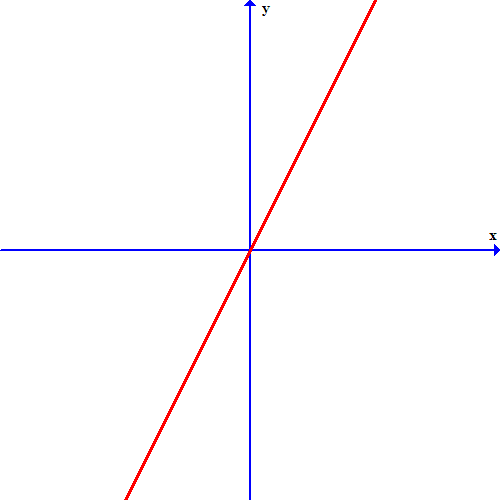#### Question 5 5. If the graph below was written in the slope-intercept form, which of the following would be true?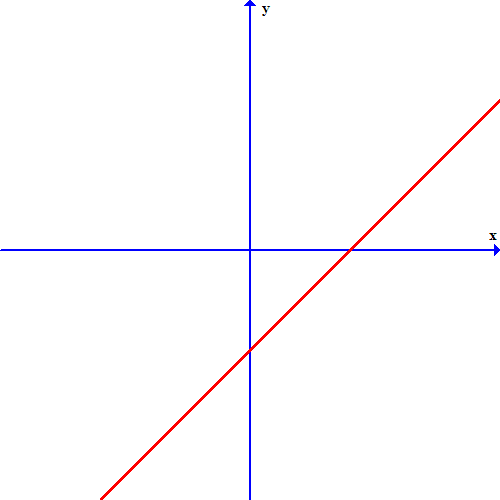### Page 3

#### Question 14 14. Which of these equations represents a line that is perpendicular to the line in the graph below?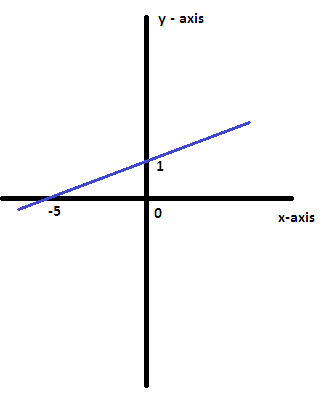### Page 4

#### Question 20 20. What is the slope-intercept form equation of the graph below?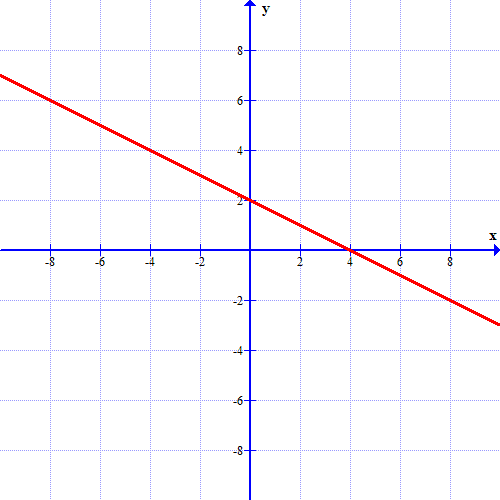### Page 5

#### Question 24 24. If the graph below has the equation Ax + By = -4, what must be true about A and B?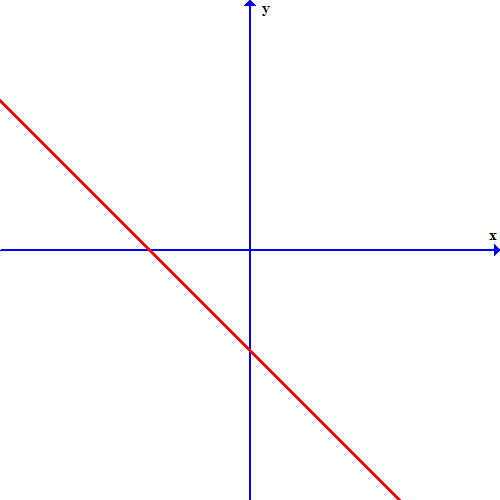### Page 6

#### Linear Equations: Precalculus Lesson Plans Chapter Exam Instructions

Choose your answers to the questions and click 'Next' to see the next set of questions. You can skip questions if you would like and come back to them later with the yellow "Go To First Skipped Question" button. When you have completed the practice exam, a green submit button will appear. Click it to see your results. Good luck!

Support